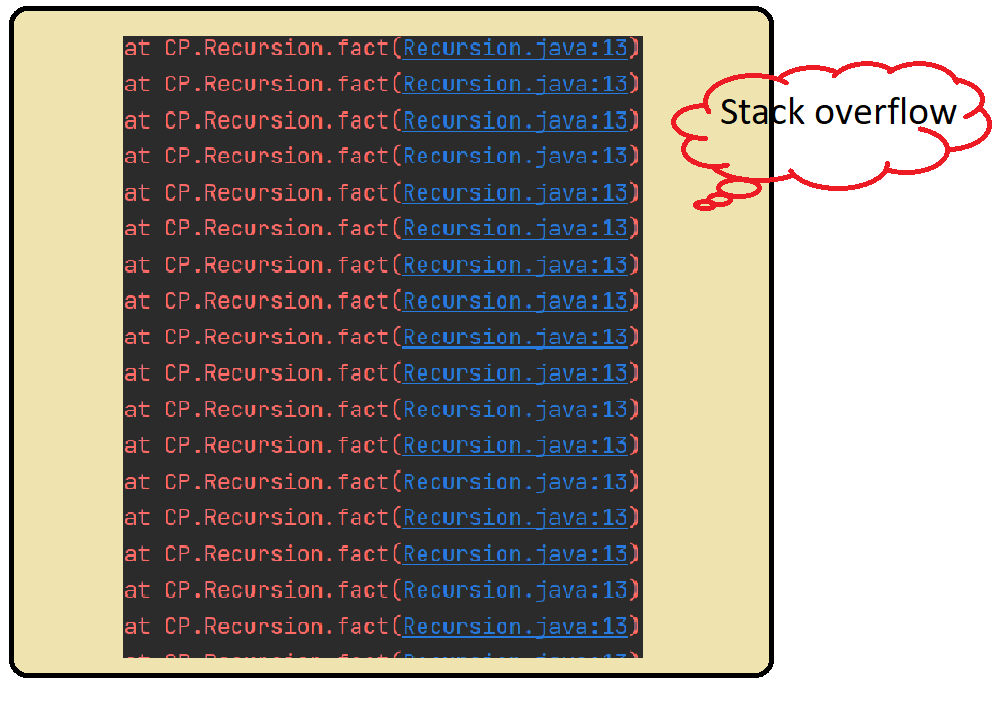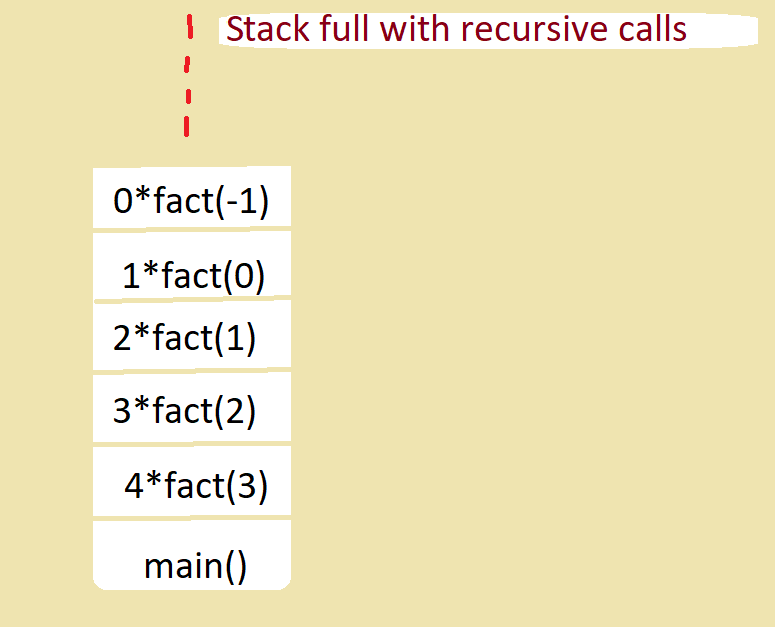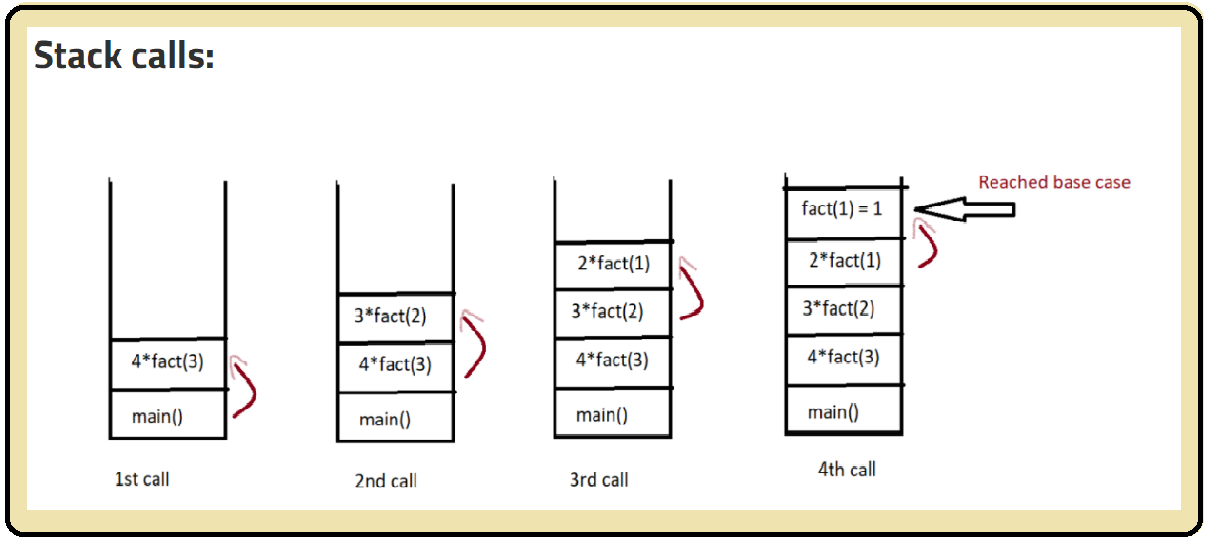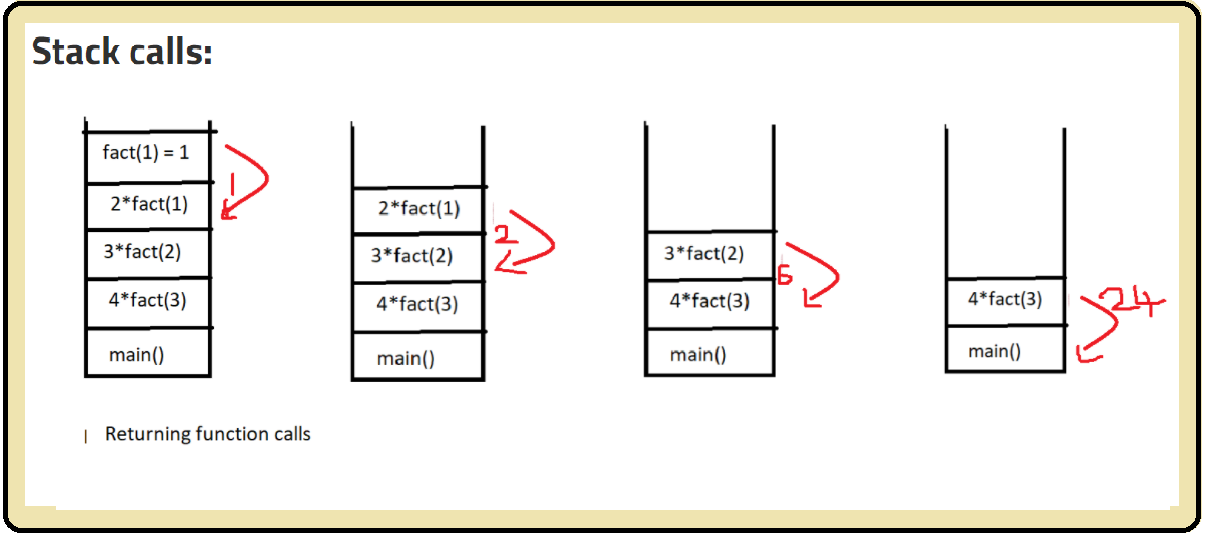# Base cases in Recursion

### Writing base cases in Recursion:

Recursion: A Function calling itself again and again directly or indirectly is called Recursion, and the function which it calls is called a recursive function, it is used in divide and conquer algorithms/techniques.

### Base cases:

The base case is also called a stopping condition for recursive calls. It is very important to have a base case for every recursive code. Without base cases the recursive calls will be made again and again many times till the Stack space allocated is filled with these recursive calls which will result in Stack overflow error, here memory gets wasted, to overcome the stack overflow error base cases plays an important role.

### Defining base cases:

Think of the least possible answer for the given question and this will be the base case

For example, if you are writing a code to print the factorial of a number then the least possible answer would be 0! Which is 1 so you will write the base case as when the given number reaches less than or equal to zero then return 1.

Now once the base is reached the recursive calls will stop and they will start returning the answer.

Usually, base cases are written in the initial steps of code, according to the given question the base cases have to be written.

Let’s see a recursive code without a base case:

Printing factorial of a number:

Code:

## Java Code

``````Printing factorial of a number:

public class Recursion {
public static void main(String[] args) {
int n = 4;
System.out.println(fact(n));
}

//function to find factorial without base case
private static int fact(int n) {
//no base case

return n*(fact(n-1));
}
}
``````

Output resulted in stack overflow error because of Not defining base caseRefer to the below picture to know  how the recursive calls are made without a base caseCode with the base condition:

As discussed the base case will be if ( n<=0) return 1

## Java Code

``````public class Recursion {
public static void main(String[] args) {
int n = 4;
System.out.println(fact(n));
}

//function to find factorial
private static int fact(int n) {

//base case
if (n<=1) return 1;

return n*(fact(n-1));
}
}
``````

Refer to the below pictures to know how recursive calls are made and how they are getting stopped and returning once they reach the base case.Special thanks to Sai bargav Nellepalli for contributing to this article on takeUforward. If you also wish to share your knowledge with the takeUforward fam, please check out this article